# ENZYMES.pptx

Lecturer
9 de May de 2023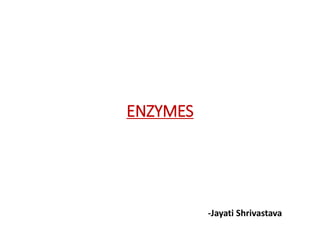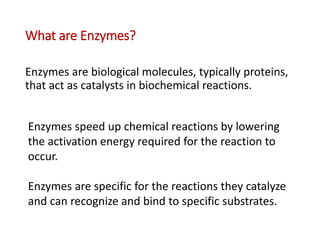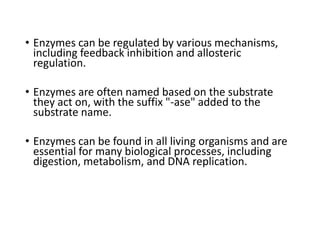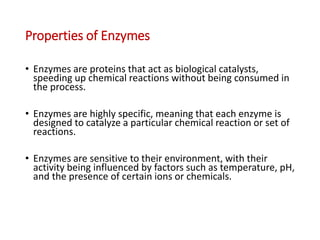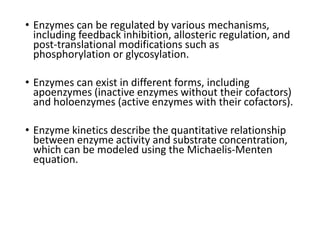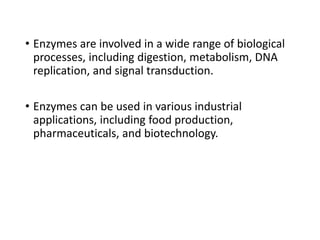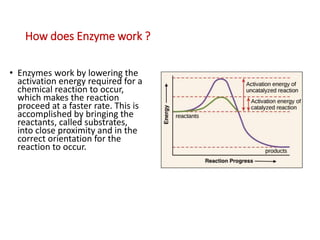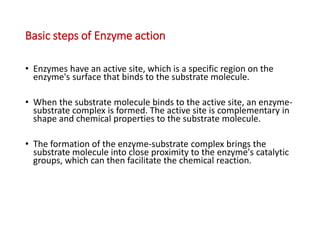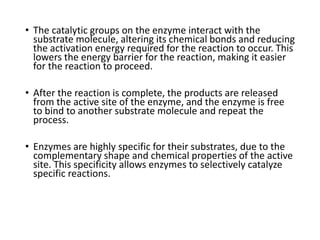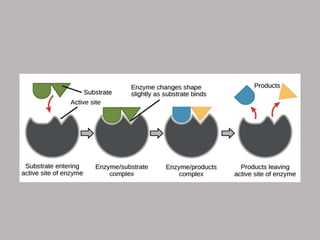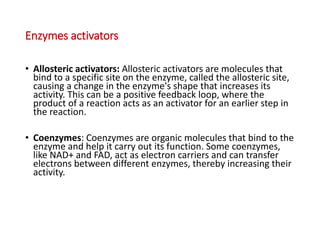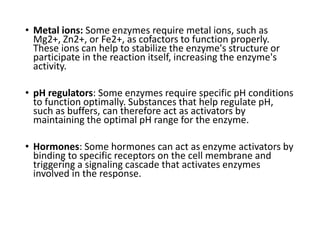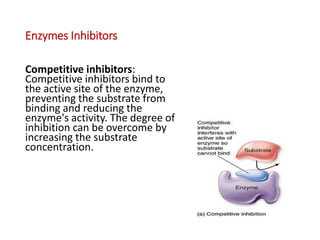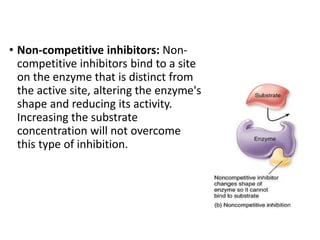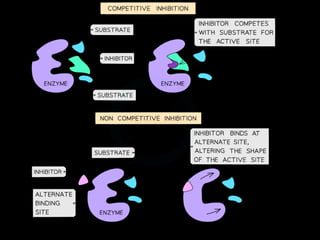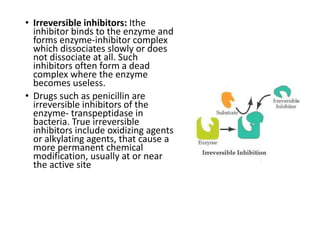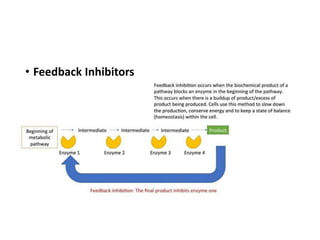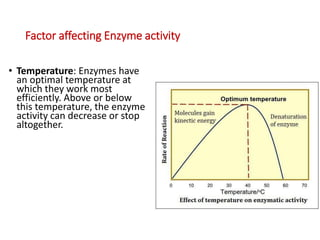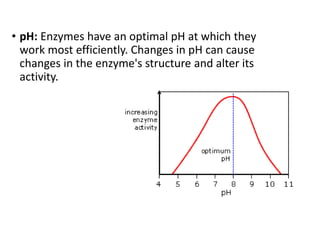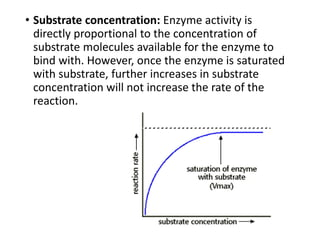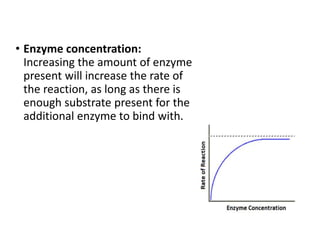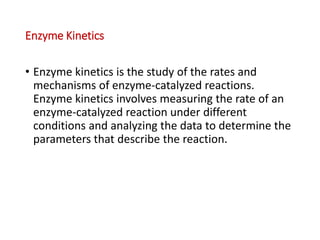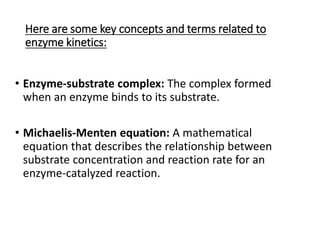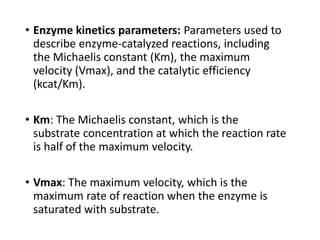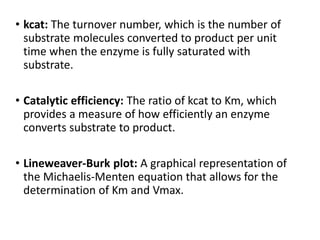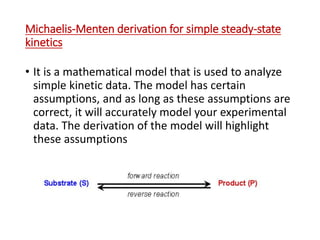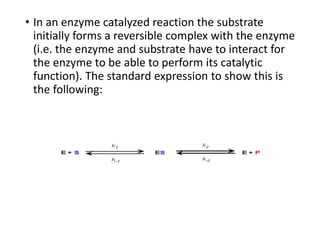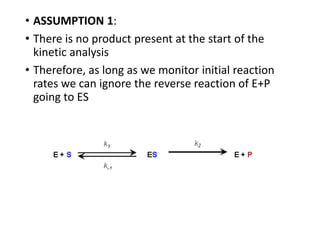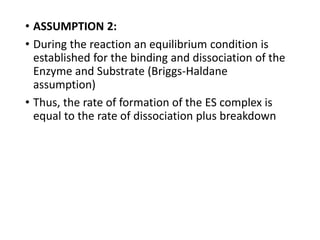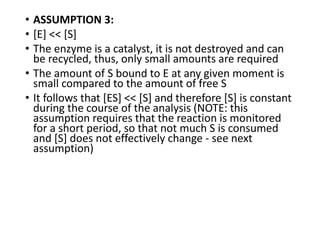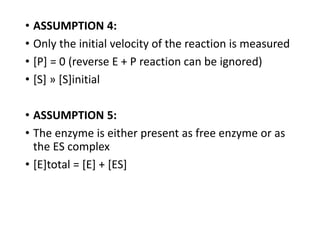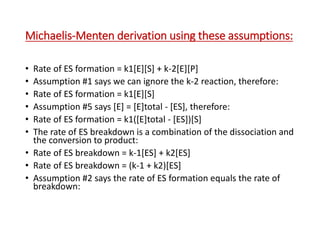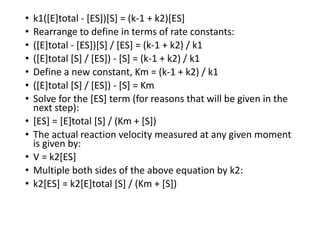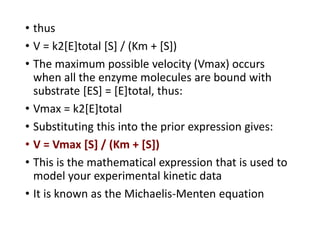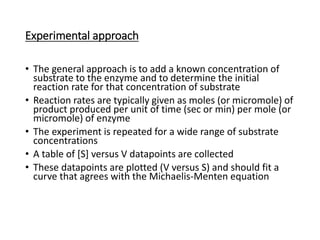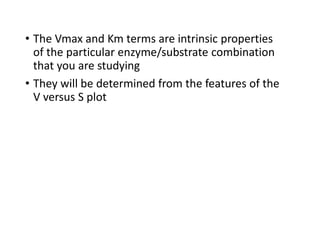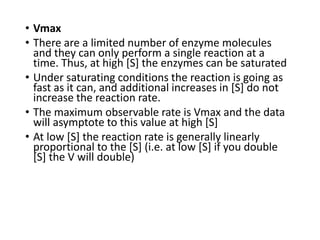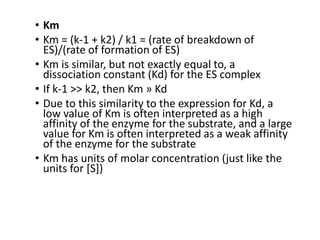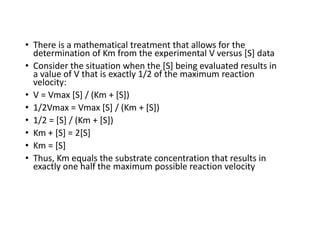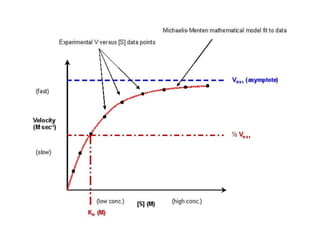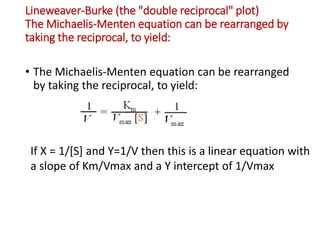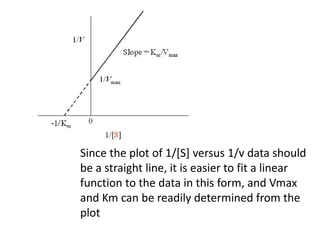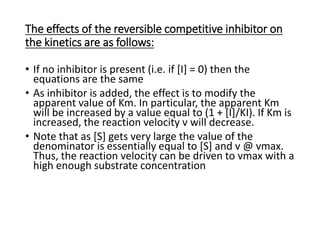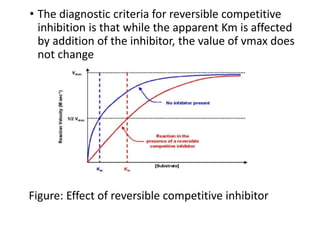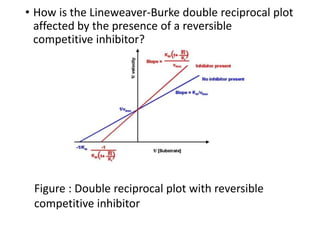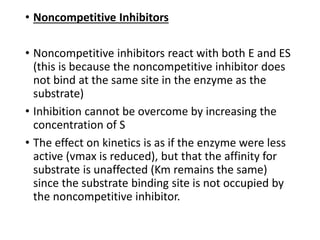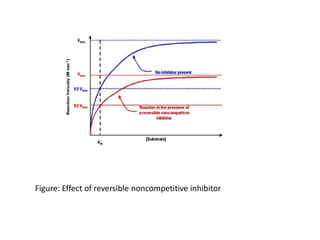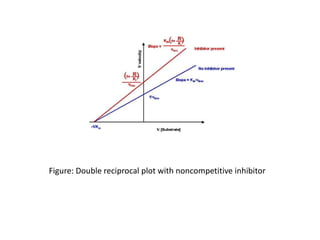1 de 48

### ENZYMES.pptx

• 1. ENZYMES -Jayati Shrivastava
• 2. What are Enzymes? Enzymes are biological molecules, typically proteins, that act as catalysts in biochemical reactions. Enzymes speed up chemical reactions by lowering the activation energy required for the reaction to occur. Enzymes are specific for the reactions they catalyze and can recognize and bind to specific substrates.
• 3. • Enzymes can be regulated by various mechanisms, including feedback inhibition and allosteric regulation. • Enzymes are often named based on the substrate they act on, with the suffix "-ase" added to the substrate name. • Enzymes can be found in all living organisms and are essential for many biological processes, including digestion, metabolism, and DNA replication.
• 4. Properties of Enzymes • Enzymes are proteins that act as biological catalysts, speeding up chemical reactions without being consumed in the process. • Enzymes are highly specific, meaning that each enzyme is designed to catalyze a particular chemical reaction or set of reactions. • Enzymes are sensitive to their environment, with their activity being influenced by factors such as temperature, pH, and the presence of certain ions or chemicals.
• 5. • Enzymes can be regulated by various mechanisms, including feedback inhibition, allosteric regulation, and post-translational modifications such as phosphorylation or glycosylation. • Enzymes can exist in different forms, including apoenzymes (inactive enzymes without their cofactors) and holoenzymes (active enzymes with their cofactors). • Enzyme kinetics describe the quantitative relationship between enzyme activity and substrate concentration, which can be modeled using the Michaelis-Menten equation.
• 6. • Enzymes are involved in a wide range of biological processes, including digestion, metabolism, DNA replication, and signal transduction. • Enzymes can be used in various industrial applications, including food production, pharmaceuticals, and biotechnology.
• 7. How does Enzyme work ? • Enzymes work by lowering the activation energy required for a chemical reaction to occur, which makes the reaction proceed at a faster rate. This is accomplished by bringing the reactants, called substrates, into close proximity and in the correct orientation for the reaction to occur.
• 8. Basic steps of Enzyme action • Enzymes have an active site, which is a specific region on the enzyme's surface that binds to the substrate molecule. • When the substrate molecule binds to the active site, an enzyme- substrate complex is formed. The active site is complementary in shape and chemical properties to the substrate molecule. • The formation of the enzyme-substrate complex brings the substrate molecule into close proximity to the enzyme's catalytic groups, which can then facilitate the chemical reaction.
• 9. • The catalytic groups on the enzyme interact with the substrate molecule, altering its chemical bonds and reducing the activation energy required for the reaction to occur. This lowers the energy barrier for the reaction, making it easier for the reaction to proceed. • After the reaction is complete, the products are released from the active site of the enzyme, and the enzyme is free to bind to another substrate molecule and repeat the process. • Enzymes are highly specific for their substrates, due to the complementary shape and chemical properties of the active site. This specificity allows enzymes to selectively catalyze specific reactions.
• 11. Enzymes activators • Allosteric activators: Allosteric activators are molecules that bind to a specific site on the enzyme, called the allosteric site, causing a change in the enzyme's shape that increases its activity. This can be a positive feedback loop, where the product of a reaction acts as an activator for an earlier step in the reaction. • Coenzymes: Coenzymes are organic molecules that bind to the enzyme and help it carry out its function. Some coenzymes, like NAD+ and FAD, act as electron carriers and can transfer electrons between different enzymes, thereby increasing their activity.
• 12. • Metal ions: Some enzymes require metal ions, such as Mg2+, Zn2+, or Fe2+, as cofactors to function properly. These ions can help to stabilize the enzyme's structure or participate in the reaction itself, increasing the enzyme's activity. • pH regulators: Some enzymes require specific pH conditions to function optimally. Substances that help regulate pH, such as buffers, can therefore act as activators by maintaining the optimal pH range for the enzyme. • Hormones: Some hormones can act as enzyme activators by binding to specific receptors on the cell membrane and triggering a signaling cascade that activates enzymes involved in the response.
• 13. Enzymes Inhibitors Competitive inhibitors: Competitive inhibitors bind to the active site of the enzyme, preventing the substrate from binding and reducing the enzyme's activity. The degree of inhibition can be overcome by increasing the substrate concentration.
• 14. • Non-competitive inhibitors: Non- competitive inhibitors bind to a site on the enzyme that is distinct from the active site, altering the enzyme's shape and reducing its activity. Increasing the substrate concentration will not overcome this type of inhibition.
• 16. • Irreversible inhibitors: Ithe inhibitor binds to the enzyme and forms enzyme-inhibitor complex which dissociates slowly or does not dissociate at all. Such inhibitors often form a dead complex where the enzyme becomes useless. • Drugs such as penicillin are irreversible inhibitors of the enzyme- transpeptidase in bacteria. True irreversible inhibitors include oxidizing agents or alkylating agents, that cause a more permanent chemical modification, usually at or near the active site
• 17. • Feedback Inhibitors
• 18. Factor affecting Enzyme activity • Temperature: Enzymes have an optimal temperature at which they work most efficiently. Above or below this temperature, the enzyme activity can decrease or stop altogether.
• 19. • pH: Enzymes have an optimal pH at which they work most efficiently. Changes in pH can cause changes in the enzyme's structure and alter its activity.
• 20. • Substrate concentration: Enzyme activity is directly proportional to the concentration of substrate molecules available for the enzyme to bind with. However, once the enzyme is saturated with substrate, further increases in substrate concentration will not increase the rate of the reaction.
• 21. • Enzyme concentration: Increasing the amount of enzyme present will increase the rate of the reaction, as long as there is enough substrate present for the additional enzyme to bind with.
• 22. Enzyme Kinetics • Enzyme kinetics is the study of the rates and mechanisms of enzyme-catalyzed reactions. Enzyme kinetics involves measuring the rate of an enzyme-catalyzed reaction under different conditions and analyzing the data to determine the parameters that describe the reaction.
• 23. Here are some key concepts and terms related to enzyme kinetics: • Enzyme-substrate complex: The complex formed when an enzyme binds to its substrate. • Michaelis-Menten equation: A mathematical equation that describes the relationship between substrate concentration and reaction rate for an enzyme-catalyzed reaction.
• 24. • Enzyme kinetics parameters: Parameters used to describe enzyme-catalyzed reactions, including the Michaelis constant (Km), the maximum velocity (Vmax), and the catalytic efficiency (kcat/Km). • Km: The Michaelis constant, which is the substrate concentration at which the reaction rate is half of the maximum velocity. • Vmax: The maximum velocity, which is the maximum rate of reaction when the enzyme is saturated with substrate.
• 25. • kcat: The turnover number, which is the number of substrate molecules converted to product per unit time when the enzyme is fully saturated with substrate. • Catalytic efficiency: The ratio of kcat to Km, which provides a measure of how efficiently an enzyme converts substrate to product. • Lineweaver-Burk plot: A graphical representation of the Michaelis-Menten equation that allows for the determination of Km and Vmax.
• 26. Michaelis-Menten derivation for simple steady-state kinetics • It is a mathematical model that is used to analyze simple kinetic data. The model has certain assumptions, and as long as these assumptions are correct, it will accurately model your experimental data. The derivation of the model will highlight these assumptions
• 27. • In an enzyme catalyzed reaction the substrate initially forms a reversible complex with the enzyme (i.e. the enzyme and substrate have to interact for the enzyme to be able to perform its catalytic function). The standard expression to show this is the following:
• 28. • ASSUMPTION 1: • There is no product present at the start of the kinetic analysis • Therefore, as long as we monitor initial reaction rates we can ignore the reverse reaction of E+P going to ES
• 29. • ASSUMPTION 2: • During the reaction an equilibrium condition is established for the binding and dissociation of the Enzyme and Substrate (Briggs-Haldane assumption) • Thus, the rate of formation of the ES complex is equal to the rate of dissociation plus breakdown
• 30. • ASSUMPTION 3: • [E] << [S] • The enzyme is a catalyst, it is not destroyed and can be recycled, thus, only small amounts are required • The amount of S bound to E at any given moment is small compared to the amount of free S • It follows that [ES] << [S] and therefore [S] is constant during the course of the analysis (NOTE: this assumption requires that the reaction is monitored for a short period, so that not much S is consumed and [S] does not effectively change - see next assumption)
• 31. • ASSUMPTION 4: • Only the initial velocity of the reaction is measured • [P] = 0 (reverse E + P reaction can be ignored) • [S] » [S]initial • ASSUMPTION 5: • The enzyme is either present as free enzyme or as the ES complex • [E]total = [E] + [ES]
• 32. Michaelis-Menten derivation using these assumptions: • Rate of ES formation = k1[E][S] + k-2[E][P] • Assumption #1 says we can ignore the k-2 reaction, therefore: • Rate of ES formation = k1[E][S] • Assumption #5 says [E] = [E]total - [ES], therefore: • Rate of ES formation = k1([E]total - [ES])[S] • The rate of ES breakdown is a combination of the dissociation and the conversion to product: • Rate of ES breakdown = k-1[ES] + k2[ES] • Rate of ES breakdown = (k-1 + k2)[ES] • Assumption #2 says the rate of ES formation equals the rate of breakdown:
• 33. • k1([E]total - [ES])[S] = (k-1 + k2)[ES] • Rearrange to define in terms of rate constants: • ([E]total - [ES])[S] / [ES] = (k-1 + k2) / k1 • ([E]total [S] / [ES]) - [S] = (k-1 + k2) / k1 • Define a new constant, Km = (k-1 + k2) / k1 • ([E]total [S] / [ES]) - [S] = Km • Solve for the [ES] term (for reasons that will be given in the next step): • [ES] = [E]total [S] / (Km + [S]) • The actual reaction velocity measured at any given moment is given by: • V = k2[ES] • Multiple both sides of the above equation by k2: • k2[ES] = k2[E]total [S] / (Km + [S])
• 34. • thus • V = k2[E]total [S] / (Km + [S]) • The maximum possible velocity (Vmax) occurs when all the enzyme molecules are bound with substrate [ES] = [E]total, thus: • Vmax = k2[E]total • Substituting this into the prior expression gives: • V = Vmax [S] / (Km + [S]) • This is the mathematical expression that is used to model your experimental kinetic data • It is known as the Michaelis-Menten equation
• 35. Experimental approach • The general approach is to add a known concentration of substrate to the enzyme and to determine the initial reaction rate for that concentration of substrate • Reaction rates are typically given as moles (or micromole) of product produced per unit of time (sec or min) per mole (or micromole) of enzyme • The experiment is repeated for a wide range of substrate concentrations • A table of [S] versus V datapoints are collected • These datapoints are plotted (V versus S) and should fit a curve that agrees with the Michaelis-Menten equation
• 36. • The Vmax and Km terms are intrinsic properties of the particular enzyme/substrate combination that you are studying • They will be determined from the features of the V versus S plot
• 37. • Vmax • There are a limited number of enzyme molecules and they can only perform a single reaction at a time. Thus, at high [S] the enzymes can be saturated • Under saturating conditions the reaction is going as fast as it can, and additional increases in [S] do not increase the reaction rate. • The maximum observable rate is Vmax and the data will asymptote to this value at high [S] • At low [S] the reaction rate is generally linearly proportional to the [S] (i.e. at low [S] if you double [S] the V will double)
• 38. • Km • Km = (k-1 + k2) / k1 = (rate of breakdown of ES)/(rate of formation of ES) • Km is similar, but not exactly equal to, a dissociation constant (Kd) for the ES complex • If k-1 >> k2, then Km » Kd • Due to this similarity to the expression for Kd, a low value of Km is often interpreted as a high affinity of the enzyme for the substrate, and a large value for Km is often interpreted as a weak affinity of the enzyme for the substrate • Km has units of molar concentration (just like the units for [S])
• 39. • There is a mathematical treatment that allows for the determination of Km from the experimental V versus [S] data • Consider the situation when the [S] being evaluated results in a value of V that is exactly 1/2 of the maximum reaction velocity: • V = Vmax [S] / (Km + [S]) • 1/2Vmax = Vmax [S] / (Km + [S]) • 1/2 = [S] / (Km + [S]) • Km + [S] = 2[S] • Km = [S] • Thus, Km equals the substrate concentration that results in exactly one half the maximum possible reaction velocity
• 41. Lineweaver-Burke (the "double reciprocal" plot) The Michaelis-Menten equation can be rearranged by taking the reciprocal, to yield: • The Michaelis-Menten equation can be rearranged by taking the reciprocal, to yield: If X = 1/[S] and Y=1/V then this is a linear equation with a slope of Km/Vmax and a Y intercept of 1/Vmax
• 42. Since the plot of 1/[S] versus 1/v data should be a straight line, it is easier to fit a linear function to the data in this form, and Vmax and Km can be readily determined from the plot
• 43. The effects of the reversible competitive inhibitor on the kinetics are as follows: • If no inhibitor is present (i.e. if [I] = 0) then the equations are the same • As inhibitor is added, the effect is to modify the apparent value of Km. In particular, the apparent Km will be increased by a value equal to (1 + [I]/KI). If Km is increased, the reaction velocity v will decrease. • Note that as [S] gets very large the value of the denominator is essentially equal to [S] and v @ vmax. Thus, the reaction velocity can be driven to vmax with a high enough substrate concentration
• 44. • The diagnostic criteria for reversible competitive inhibition is that while the apparent Km is affected by addition of the inhibitor, the value of vmax does not change Figure: Effect of reversible competitive inhibitor
• 45. • How is the Lineweaver-Burke double reciprocal plot affected by the presence of a reversible competitive inhibitor? Figure : Double reciprocal plot with reversible competitive inhibitor
• 46. • Noncompetitive Inhibitors • Noncompetitive inhibitors react with both E and ES (this is because the noncompetitive inhibitor does not bind at the same site in the enzyme as the substrate) • Inhibition cannot be overcome by increasing the concentration of S • The effect on kinetics is as if the enzyme were less active (vmax is reduced), but that the affinity for substrate is unaffected (Km remains the same) since the substrate binding site is not occupied by the noncompetitive inhibitor.
• 47. Figure: Effect of reversible noncompetitive inhibitor
• 48. Figure: Double reciprocal plot with noncompetitive inhibitor